The Transition from Far to Near Field calculated by Vortex Physics | OMICS International
Fluid Mechanics: Open Access

# The Transition from Far to Near Field calculated by Vortex Physics

Konstantin Meyl*

Faculty of Computer and Electrical Engineering, Furtwangen University, TVillingen-Schwenningen, Germany

*Corresponding Author:
Konstantin Meyl
Faculty of Computer and Electrical Engineering
Furtwangen University, TZS, Erikaweg 32
D-78048, Villingen-Schwenningen, Germany
Tel: +4977203070
E-mail: [email protected]

Received Date: October 21, 2015; Accepted Date: December 20, 2015; Published Date: January 01, 2016

Citation: Meyl K (2016) The Transition from Far to Near Field calculated by Vortex Physics. Fluid Mech Open Acc 3:122. doi:10.4172/fmoa.1000122

Copyright: © 2016 Meyl K. This is an open-access article distributed under the terms of the Creative Commons Attribution License, which permits unrestricted use, distribution, and reproduction in any medium, provided the original author and source are credited.

Visit for more related articles at Fluid Mechanics: Open Access

#### Abstract

Continuing the contribution about “wireless power transmission by scalar waves”, presented in Moscow 2012, the paper from PIERS Stockholm 2013 goes deeper, explaining the different types and properties of waves described by the wave equation. Starting with the wave description of Maxwell an extended version of the Laplace equation is derived, expanding the standard derivations of the near field, showing how to influence the zone, where the longitudinal wave parts occur. We come to the conclusion that the near field zone is enlarging, if the longitudinal parts of a wave or the antenna voltage are accelerated. This relationship is essential for the wireless transmission of energy. In addition this exciting new result could be tested experimentally. The simple experiment has been demonstrated more often. Only by changing the antenna design, it was shown how both, the speed of propagation and the near field are enlarging proportional to each other. In all practical applications, as discussed in different congress Proceedings, the extended near field is the key of success. This paper contents the calculation of the transition from near field to far field of an antenna, resp. from field vortex propagation, as a so called scalar wave, to an electromagnetic wave.

#### Keywords

Vortex; Wireless power; Transmission; Conductivity

#### Introduction (A Wave Damped by Potential Vortices)

The wave equation, as it has been derived from the field equations according to Maxwell, will be the starting-point [1,2]. For the case of a wave propagation in air or in vacuum, if no electric conductivityis present (σ = 0 and div E = 0), the fundamental field equation is reduced to the two parts: the description of the electromagnetic wave and the potential vortex as a damping term :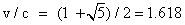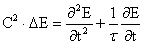with the relaxation time constant τ (1)

Now a solution of this partial differential equation is sought. This only succeeds for a very particular course of spatial and temporal field.

If a wave for a field perturbation rolls up to a vortex, which has been worked out as a model concept , then the field oscillation continues to run with c, the speed of light, but this time in circles. With this consideration the relation between the angular velocity resp. the time constants and the radius of the circular vortex has been described (τ = r/c), based on the distribution of the field of a dipole in the so called near field zone (Figure 1).

#### Calculation

In the case of a perturbation this wave rolls up to a vortex, with

• The swirl velocity c = ω⋅r, and

• The angular velocity ω = 1/τ = c/r

v(x(t)) = dx/dt is the non-accelerated velocity of propagation of a vortex as a longitudinal wave in direction of the E-field: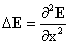• v(x(t)) = vx points in the x-direction radially to the outside. For the derivations of the approach E (x(t)) = E (t)⋅e–x/r of the field vector is valid: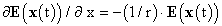and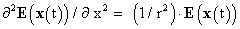(2)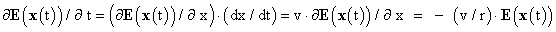and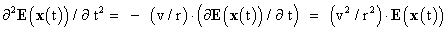(3)

Thus the field equation (1), defined in space and time, can be converted into an equation determined in v and x: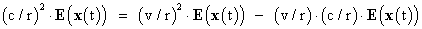(4)

There remains a quadratic equation to determine the velocity v: c2 = v2 - v⋅c resp.:v2 - v⋅c - c2 = 0

From the two solutions of the quadratic equation only the one with positive velocity should be considered: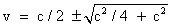and(6)

and that would be 1.618 times the speed of light. If we subtract 1 from this value or form the reciprocal value, then in both cases the factor Φ = 0.618 results, which is called the Golden Proportion:

c/v = Φ = 0.618 according to that is: 1 + Φ = 1.618 = 1/Φ = 1/0.618 (7)

Behind this mathematically won result is hiding a deeper physical meaning. Obviously nothing can hinder a longitudinal wave and its vortices to be slower or faster than just with v = 1.618⋅c .

#### Interpretation of the Calculated Result

Let us take the case that v = c, for which there exist calculations in some textbooks . As a result gets out that the longitudinal parts decrease very quickly and already can be neglected after λ/2π. Thus the interpretation of the near-field zone of an antenna says that within one sixth of the wavelength most of the vortices have decayed.

For larger velocities, as Tesla has produced already 100 years ago, the stability of the vortices increases. From a high-frequency technical viewpoint this is the domain of the antenna noise, the domain of instable oscillating noise vortices.

This domain stretches from c ≤ v to v ≤ 1.618⋅c, the boundary case, where a spontaneous conversion of the wave into the vortex is possible. The Golden Proportion describes the stability limit, where the field vortices after they have been formed, don’t decay anymore. From this velocity on the antenna resp. radiation source hasn’t got a limited nearfield zone anymore, since that now reaches to infinity. Only from this distinct velocity on we give the field vortices the name “neutrino”. This is possible and acceptable, as all well known properties of an oscillating neutrino are covered (Table 1).

In the domain Vortex decay Description
v = c Within λ/2π Near-field zone
v < 1.618.c Only later Noise
v = 1.618.c Stability limit Direct conversion
v > 1.618.c Stable, no decay Neutrino radiation

Table 1: In the ideal case at 1.618 times the speed of light at the stability limit, the natural and spontaneous conversion from vortex to wave and vice versa occurs. Scalar wave antennas have their optimum degree of effectiveness in the Golden Proportion.

#### The Golden Proportion

Whereas traditional antennas, which should emit electromagnetic waves, are optimized for signals with the speed of light, do scalar wave antennas have their optimum degree of effectiveness in the Golden Proportion. Thereby the goal of an emission of oscillating ring-like vortices is pursued.

Vortices, which with a velocity of propagation of v ≥ 1.618⋅c remain stable and make possible a spatially unlimited transmissionof energy and information by scalar waves.

The velocity of propagation v is calculated from the product of wavelength times frequency: v = λ ⋅ f.

If the frequency of a transmitter is prescribed, then the geometry of the transmitting antenna decides on the degree of effectiveness of the antenna and on how many parts of transverse waves are emitted in relation to longitudinal waves. In the case of a broadcast antenna usually λ or λ/2 is chosen. For a scalar wave however the optimum is reached with:

λvortex = 1.618 ⋅ λwave = 1.618 ⋅ c/f (8)

With that the electrical problem becomes a geometrical one, if it concerns the use of scalar waves. Crucial is the antenna geometry, and the Golden Proportion provides the necessary constructional instruction. Were the buildings in antiquity, which were constructed according to the Golden Proportion, technical facilities for scalar waves? Did the builders have physically explainable and mathematically provable guidelines? .

Consequences (Neutrino with more than Speed of Light?)

The neutrino physicists proceed from the assumption that their particles are on the way with a speed somewhat less than the speed of light c. This contradicts the observation according to which black holes should represent strong sources of neutrinos, which are black only for the reason that no particle radiation is able to escape them, which is on the way with c or even slower. If a black hole does hurl neutrino radiation into space, than that must be considerably faster than c, as normal neutrino physicists still by no means can imagine it today.

But the neutrino radiation only can be detected after it has been slowed down to a value, which is smaller than c. The “measurement” of a rest mass could be a classical measurement error. As long as a neutrino on the way to us still is faster than the light, the average of its mass is generally zero. The effective value of the mass of a neutrino is however considerable. Only it is able to give account for the sought-for dark matter, as far as it must exist in the today supposed form anyway.

The Tesla radiation, that the discoverer Nikola Tesla already in own experiments had found out, is faster than light . Since this Tesla radiation according to the description is identical with the neutrino radiation, since it so to say forms a subset, we will call neutrino radiation all the scalar waves, which are faster than 1,618 times the speed of light. This stretches from the weak radiation at low frequencies up to the strong neutrino radiation of cosmic origin. But the hardness of the radiation does not only increase with the frequency, it in particular increases with the velocity.

Longitudinal waves are propagating in the direction of a field pointer which is oscillating. As a consequence the speed is oscillating as well and never constant. Thus there is no limitation to the speed of light (Figure 2).

From other areas, for instance from flow dynamics or for body sound is generally known that both wave parts exist, the longitudinal and transverse parts. In the case of a propagation through the earth, like for an earthquake, both parts are received and utilized. Because their propagation is differently fast, the faster oscillations arrive first and that are the longitudinal ones. From the time delay with which the transverse waves arrive at the measurement station, the distance to the epicentre of the quake is determined by means of the different velocity of propagation. For geophysicists this tool is part of everyday knowledge.

Obviously all particles, propagating as a longitudinal wave, move quicker than the transverse wave part, produced by the same antenna at the same time.

#### References

Select your language of interest to view the total content in your interested language

### Article Usage

• Total views: 9347
• [From(publication date):
July-2016 - Dec 07, 2019]
• Breakdown by view type
• HTML page views : 9141Can't read the image? click here to refresh